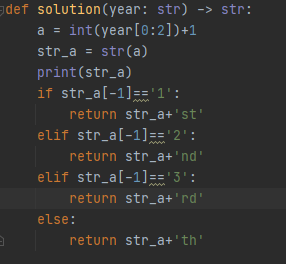# 【每日一题20220223】第几世纪给定一个四位数组成的字符串表示年份year，请编写一个函数solution，返回它代表的是第几世纪标记，由数字和小写英文字母缩写组成。

``````def solution(year: str) -> str:

assert solution("2022") == "21st"
assert solution("2154") == "22nd"
assert solution("2259") == "23rd"
assert solution("1234") == "13th"
``````
``````def solution(year: str) -> str:
m = int(year) // 100
if (m+1) % 10 == 1:
return "".join(str(m+1)+'st')
elif (m+1) % 10 == 2:
return "".join(str(m+1)+'nd')
elif (m+1) % 10 == 3:
return "".join(str(m+1)+'rd')
else:return ''.join(str(m+1)+'th')

assert solution("2022") == "21st"
assert solution("2154") == "22nd"
assert solution("2259") == "23rd"
assert solution("1234") == "13rd"
assert solution('1456') == "15th"
``````
``````def solution(year: str) -> str:
num_base = int(int(year) / 100)
digit_num = int(str(num_base)[-1])
case_num = int(str(num_base))
y = {
0 : str(num_base + 1) + "st",
1 : str(num_base + 1) + "nd",
2 : str(num_base + 1) + "rd",
}
if case_num >= 2 and digit_num in y.keys():
return y.get(digit_num)
else:
return str(num_base + 1) + "th"

assert solution("2022") == "21st"
assert solution("2154") == "22nd"
assert solution("2259") == "23rd"
assert solution("1234") == "13th"
``````

11-19 应该都是th结尾的

2 Likes

``````def solution(year: str) -> str:
shiji = int(year)//100 + 1
if shiji >= 20:
if str(shiji)[-1] == "1":
return str(shiji)+"st"
elif str(shiji)[-1] == "2":
return str(shiji) + "nd"
else:
return str(shiji) + "rd"
else:
return str(shiji) + "th"
``````

``````
def solution(year: str) -> str:
cen = int(year[:2]) + 1

if cen % 10 == 1:   th = 'st'
elif cen % 10 == 2: th = 'nd'
elif cen % 10 == 3: th = 'rd'
else:               th = 'th'

if cen // 10 == 1:
th = 'th'
return str(cen) + th

assert solution("2022") == "21st"
assert solution("2154") == "22nd"
assert solution("2259") == "23rd"
assert solution("1234") == "13th"

``````
``````def solution(year: str) -> str:
y = int(year[0:2]) + 1
if int(year) >= 2:
if y % 10 == 1:
return str(y) + 'st'
elif y % 10 == 2:
return str(y) + 'nd'
elif y % 10 == 3:
return str(y) + 'rd'
else:
return str(y) + 'th'
else:
return str(y) + 'th'

assert solution("2022") == "21st"
assert solution("2154") == "22nd"
assert solution("2259") == "23rd"
assert solution("1234") == "13th"
``````
``````def solution(year: str) -> str:
part1 = int(int(year) / 100) # 取整
part2 = int(year) % 100   # 取余
if part2 != 0:
part1 += 1
if str(part1)[-1] == '1':
return str(part1)+'st'
elif str(part1)[-1] == '2':
return str(part1) + 'nd'
elif str(part1)[-1] == '3':
return str(part1) + 'rd'
else:
return str(part1) + 'nd'

assert solution("2022") == "21st"
assert solution("2154") == "22nd"
assert solution("2259") == "23rd"
assert solution("1234") == "13rd"``````参考大佬们的说法，补上了11-19的逻辑

``````def solution(year: str) -> str:
century_num = int(int(year)/100) + 1
last_num = century_num % 10
if last_num == 1:
abbr_word = "st"
elif last_num == 2:
abbr_word = "nd"
elif last_num == 3:
abbr_word = "rd"
else:
abbr_word = "th"
if century_num // 10 == 1:
abbr_word = 'th'
return str(century_num) + abbr_word

assert solution("2022") == "21st"
assert solution("2154") == "22nd"
assert solution("2259") == "23rd"
assert solution("1234") == "13th"

``````
``````def solution(year):
datas = {1:'st', 2:'nd', 3:'rd'}
end = int(year[-2:])
century = int(year[:2])

if end > 0:
century = century + 1
else:
century = century

results = ''
th_case = [x for x in range(11,20)]
if century not in th_case and int(str(century)[-1])in datas:
results = '%s%s' % (century, datas[int(str(century)[-1])])
else:
results = '%s%s' % (century, 'th')
return results
``````
``````
def solution(year: str) -> str:
shiji=str(int(year[:2])+1)
if int(shiji)>=20:
if shiji[-1]=='1':
return shiji+'st'
elif shiji[-1]=='2':
return shiji + 'nd'
elif shiji[-1]=='3':
return shiji + 'rd'
else:
return shiji + 'th'
``````
``````        def solution(year: str) -> str:
century = int(year) // 100 + 1
if 3 < century < 20:return str(century) + 'th'  #看了评论,有大佬,加了这句
suffix = 'st' if century % 10 == 1 else 'nd' if century % 10 == 2 else 'rd' if century % 10 == 3 else 'th'
return str(century) + suffix
``````